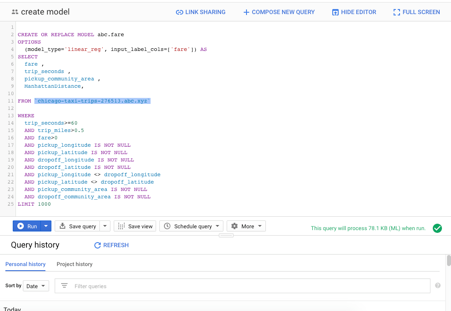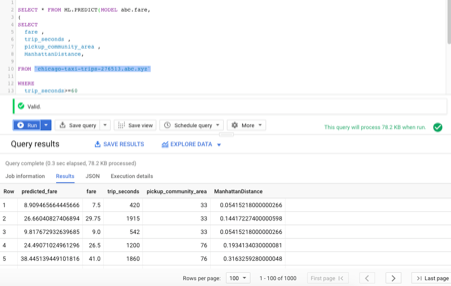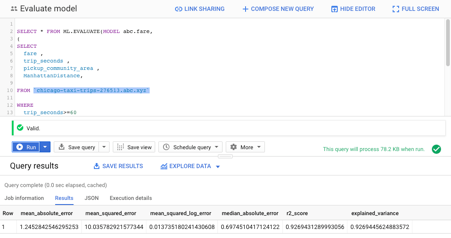# Predicting Chicago Taxi Fares | Big Data

### Hello World,

TLDR; Principal Component Analysis to find relationship between multiple variables for Chicago Taxi Fare dataset.

This assignment was given by Prof Patrick Healy at the University of Limerick for a Big Data and Visualisation module (CS6502)

In Lab 11, you have learned how to create and evaluate a machine learning model, then predict using the model. In this project you are asked to practice the skills on a different dataset – Chicago Taxi Trips, this dataset includes taxi trips from 2013 to the present, reported to the City of Chicago.

1. use the python scikit-learn to investigate the similarities and relationships that PCA can provide. It will not be possible for you to analyze the entire data set in this way so you should perform your analysis on *the first 1000 rows* of the table. Ideally the output of this phase should guide the direction you take in the second, ML, part of the assignment. You will need to decide which columns should be part of your analysis and which should be ignored. See some of the tutorial links we have posted in the lecture slides for help in deciding what are appropriate columns to consider.

2. based on the information you gained from step 1, create a model to predict the taxi fare1(“fare” column in the dataset).

Note that you may need to clean the data, pick a list of features (feature engineering), and then design your model. Please email your zipped solution pack to the lecturer by the end of week 14 with subject “cs6502:ml proj”.

Note that your solution pack should contain
-the query you use to selected the first 1000 rows
-key steps for your PCA (setup, command, etc)
-the sql for model creation
-the sql to evaluate the model
-the SQL to predict using the model above
-link of the BigQuery commands you composed
-screenshot of the model evaluation report

You will be assessed on the quality of the information you glean and feed into the ML part and how you present this to us. Again, see how this is done from the tutorials. (Note, as the tutorials make clear the R statistical package has very good support for this type of analysis. If you wish to take this task on using R then this will be ok, too.)

### Solutions

The following document contains a solution pack:

-the query you use to selected the first 1000 rows

-key steps for your PCA (setup, command, etc)

-the sql for model creation

-the sql to evaluate the model

-the sql to predict using the model above

-link of the BigQuery commands you composed

-screenshot of the model evaluation report

The query you use to selected the first 1000 rows

SELECT  fare ,  EXTRACT(DAYOFWEEK from trip_start_timestamp )AS dayofweek,  EXTRACT(HOUR from trip_start_timestamp ) AShourofday,  trip_miles ,  trip_seconds ,  pickup_longitude ,   pickup_latitude ,   dropoff_longitude ,   dropoff_latitude ,  pickup_community_area ,  dropoff_community_area ,  trip_start_timestampFROM  bigquery-public-data.chicago_taxi_trips.taxi_tripsWHERE   trip_seconds>=60  AND trip_miles>0.5  AND fare>0  AND pickup_longitude IS NOT NULL  AND pickup_latitude IS NOT NULL  AND dropoff_longitude IS NOT NULL  AND dropoff_latitude IS NOT NULL  AND pickup_longitude <>dropoff_longitude  AND pickup_latitude <> dropoff_latitude  AND pickup_community_area IS NOT NULL  AND dropoff_community_area IS NOT NULL  ANDMOD(ABS(FARM_FINGERPRINT(CAST(trip_start_timestamp AS STRING))), 5000)= 1LIMIT1000

Key steps for your PCA (setup, command, etc)

PCA and Data exploration, cleaning, feature

Importing

import pandas as pd import numpy as npimport matplotlib.pyplot as pltfrom sklearn.decomposition import PCAfrom sklearn.preprocessing import StandardScaler%matplotlib inline# Pandas display optionspd.set_option('display.float_format', lambda x: '%.3f' % x)# Set random seed RSEED = 10# Visualizationsimport matplotlib.pyplot as plt%matplotlib inlineplt.style.use('fivethirtyeight')import seaborn as snspalette = sns.color_palette('Paired', 10)

Importing data set (random 1000 rows from Chicago Taxi Fare dataset)

url = "/content/results-20200510-190212.csv"
df = pd.read_csv(url)df.head()

08.6504232.600420-87.64941.923-87.63341.900782015-07-22 23:45:00 UTC
18.4504232.300540-87.61341.892-87.64341.8798282015-07-22 23:45:00 UTC
27.2504231.800480-87.61941.891-87.64341.8798282015-07-22 23:45:00 UTC
36.0504231.300300-87.62941.900-87.61341.892882015-07-22 23:45:00 UTC
47.0504231.600420-87.63841.893-87.61941.891882015-07-22 23:45:00 UTC

df.describe()
count1000.0001000.0001000.0001000.0001000.0001000.0001000.0001000.0001000.0001000.0001000.000
mean13.2573.21716.2303.926824.164-87.65641.894-87.64341.89624.64320.044
std11.6971.3184.6795.090719.8860.0720.0400.0420.03719.62315.238
min4.4501.00013.0000.51060.000-87.91441.713-87.91441.7061.0001.000
25%6.7501.00013.0001.177420.000-87.64541.879-87.64841.8818.0008.000
50%8.7504.00013.0001.900633.500-87.63241.892-87.63241.89228.0008.000
75%13.3004.00023.0003.900960.000-87.62141.900-87.62141.90132.00032.000
max124.2504.00023.00064.40013620.000-87.58342.010-87.57342.01077.00077.000
plt.figure(figsize = (10, 6))sns.distplot(df['fare']);plt.title('Distribution of Fare');Binning

# Bin the fare and convert to stringdf['fare-bin'] = pd.cut(df['fare'], bins = list(range(0, 50, 5))).astype(str)# Uppermost bindf.loc[df['fare-bin'] == 'nan', 'fare-bin'] = '[45+]'# Adjust bin so the sorting is correctdf.loc[df['fare-bin'] == '(5.0, 10.0]', 'fare-bin'] = '(05.0, 10.0]'# Bar plot of value countsdf['fare-bin'].value_counts().sort_index().plot.bar(color = 'b', edgecolor = 'k');plt.title('Fare Binned');Lat Long Pickup drop distribution

fig, axes = plt.subplots(1, 2, figsize = (20, 8), sharex=True, sharey=True)axes = axes.flatten()# Plot Longitude (x) and Latitude (y)sns.regplot('pickup_longitude', 'pickup_latitude', fit_reg = False,data = df.sample(100, random_state = RSEED), ax = axes);sns.regplot('dropoff_longitude', 'dropoff_latitude', fit_reg = False,data = df.sample(100, random_state = RSEED), ax = axes);axes.set_title('Pickup Locations')axes.set_title('Dropoff Locations');# Absolute difference in latitude and longitudedf['abs_lat_diff'] = (df['dropoff_latitude'] - df['pickup_latitude']).abs()df['abs_lon_diff'] = (df['dropoff_longitude'] - df['pickup_longitude']).abs()
sns.lmplot('abs_lat_diff', 'abs_lon_diff', fit_reg = False,data = df.sample(900, random_state=RSEED));plt.title('Absolute latitude difference vs Absolute longitude difference');sns.lmplot('abs_lat_diff', 'abs_lon_diff', hue = 'fare-bin', size = 8, palette=palette,fit_reg = False, data = df.sample(900, random_state=RSEED));plt.title('Absolute latitude difference vs Absolute longitude difference');It does seem that the rides with a larger absolute difference in both longitude and latitude tend to cost more. To capture both differences in a single variable, we can add up the two differences in latitude and longitude and also find the square root of the sum of differences squared. The former feature would be called the Manhattan distance - or l1 norm - and the latter is called the Euclidean distance - or l2 norm. Both of these distances are specific examples of the general Minkowski distance.

Manhattan and Euclidean Distance

The Minkowski Distance between two points is expressed as:

𝐷(𝑋,𝑌)=(𝑖=1𝑛|𝑥𝑖𝑦𝑖|𝑝)1/𝑝if p = 1, then this is the Manhattan distance and if p = 2 this is the Euclidean distance. You may also see these referred to as the l1 or l2 norm where the number indicates p in the equation.

I should point out that these equations are only valid for actual distances in a cartesian coordinate system and here we only use them to find relative distances. To find the actual distances in terms of kilometers, we have to work with the latitude and longitude geographical coordinate system. This will be done later using the Haversine formula.

def minkowski_distance(x1, x2, y1, y2, p):    return ((abs(x2 - x1) ** p) + (abs(y2 - y1)) ** p) ** (1 / p)
# Create a color mapping based on fare binscolor_mapping = {fare_bin: palette[i] for i, fare_bin in enumerate(df['fare-bin'].unique())}color_mapping
df['color'] = df['fare-bin'].map(color_mapping)plot_data = df.sample(100, random_state = RSEED)
df['manhattan'] = minkowski_distance(df['pickup_longitude'], df['dropoff_longitude'],df['pickup_latitude'], df['dropoff_latitude'], 1)# Calculate distribution by each fare binplt.figure(figsize = (12, 6))for f, grouped in df.groupby('fare-bin'):sns.kdeplot(grouped['manhattan'], label = f'{f}', color = list(grouped['color']));plt.xlabel('degrees'); plt.ylabel('density')plt.title('Manhattan Distance by Fare Amount');df['euclidean'] = minkowski_distance(df['pickup_longitude'], df['dropoff_longitude'],df['pickup_latitude'], df['dropoff_latitude'], 2)# Calculate distribution by each fare binplt.figure(figsize = (12, 6))for f, grouped in df.groupby('fare-bin'):sns.kdeplot(grouped['euclidean'], label = f'{f}', color = list(grouped['color']));plt.xlabel('degrees'); plt.ylabel('density')plt.title('Euclidean Distance by Fare Amount');These features do seem to have some differences between the different fare amounts, so they might be helpful in predicting the fare.

grouped = df.groupby('fare-bin')['euclidean'].agg(['mean', 'count'])grouped.sort_index(ascending=True)
meancount
fare-bin
(0.0, 5.0]0.01321
(05.0, 10.0]0.020578
(10.0, 15.0]0.043196
(15.0, 20.0]0.06954
(20.0, 25.0]0.08718
(25.0, 30.0]0.16131
(30.0, 35.0]0.17321
(35.0, 40.0]0.23619
(40.0, 45.0]0.27936
[45+]0.27326
df.groupby('fare-bin')['euclidean'].mean().plot.bar(color = 'b');plt.title('Average Euclidean Distance by Fare Bin');There is a very clearly relationship between the fare bin and the average distance of the trip! This should give us confidence that this feature will be useful to a model.

corrs = df.corr()corrs['fare'].plot.bar(color = 'g', figsize = (10, 10));plt.title('Correlation with Fare Amount');corrs = df.corr()plt.figure(figsize = (14, 14))sns.heatmap(corrs, annot = True, vmin = -1, vmax = 1, fmt = '.3f', cmap=plt.cm.PiYG_r);the basic idea when using PCA as a tool for feature selection is to select variables according to the magnitude (from largest to smallest in absolute values) of their coefficients (loadings)

from sklearn.preprocessing import StandardScalerfeatures = ['manhattan', 'dayofweek', 'hourofday', 'trip_seconds', 'pickup_longitude', 'pickup_latitude', 'dropoff_longitude', 'dropoff_latitude', 'pickup_community_area', 'dropoff_community_area']# Separating out the featuresx = df.loc[:, features].values# Separating out the targety = df.loc[:,['fare']].values# Standardizing the featuresx = StandardScaler().fit_transform(x)x
features = x.Tcovariance_matrix = np.cov(features)print(covariance_matrix)
eig_vals, eig_vecs = np.linalg.eig(covariance_matrix)
print('Eigenvectors \n%s' %eig_vecs)
print('\nEigenvalues \n%s' %eig_vals)
eig_vals / sum(eig_vals)

Standardize the Data Since PCA yields a feature subspace that maximizes the variance along the axes, it makes sense to standardize the data, especially, if it was measured on different scales. let us continue with the transformation of the data onto unit scale (mean=0 and variance=1), which is a requirement for the optimal performance of many machine learning algorithms.

pca = PCA()features = pca.fit_transform(x)
explained_variance = pca.explained_variance_ratio_explained_variance
def myplot(score,coeff,labels=None):xs = score[:,0]ys = score[:,1]n = coeff.shapescalex = 1.0/(xs.max() - xs.min())scaley = 1.0/(ys.max() - ys.min())plt.scatter(xs * scalex,ys * scaley, c = y)for i inrange(n):plt.arrow(0, 0, coeff[i,0], coeff[i,1],color = 'r',alpha = 0.5)if labels isNone:plt.text(coeff[i,0]* 1.15, coeff[i,1] * 1.15, "Var"+str(i+1), color = 'g', ha = 'center', va = 'center')else:plt.text(coeff[i,0]* 1.15, coeff[i,1] * 1.15, labels[i], color = 'g', ha = 'center', va = 'center')plt.xlim(-1,1)plt.ylim(-1,1)plt.xlabel("PC{}".format(1))plt.ylabel("PC{}".format(2))plt.grid()#Call the function. Use only the 2 PCs.myplot(features[:,0:2],np.transpose(pca.components_[0:2, :]))plt.show()pca.explained_variance_ratio_
cumsum = np.cumsum(pca.explained_variance_ratio_)
%matplotlib inlineimport matplotlib.pyplot as pltplt.xlabel("number of components")plt.ylabel("Cumulative explained variance")plt.plot(cumsum,'--o')print(abs( pca.components_ ))

Here, pca.components_ has shape [n_components, n_features]. Thus, by looking at the PC1 (First Principal Component) which is the first row: the 1st, 4th, 5th and 9th variables are most important. which in this case are 'manhattan distance', 'trip_seconds', 'pickup_longitude', 'pickup_community_area'.

However, the pickup longitude is something that is a raw unprocessed feature and the manhattan distance is brought to use after feature procesing so we will be considering that.

NOTE: We are not going to be taking the trip miles because a) it is usually not available at the beginning of the journey AND b) it is highly corelated with the manhattan distance, and we should not taken multiple variables that are highly corelated to each other in order to decrease generalisation error.

Now lets move onto the ML part on Big Query in GCP

The sql for model creation https://console.cloud.google.com/bigquery?sq=1085073370079:ac74aed7fd1b4bdc8f6b85a60dfa5bc6Model Test/PredictEvaluate
Model -
the sql to evaluate the modelReferences:

https://stackoverflow.com/questions/40120696/trying-to-get-the-euclidean-distance-through-a-query